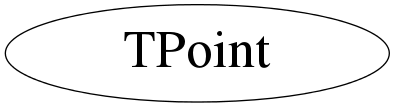# class TPoint

```
TPoint

Don't add in dictionary since that will add a virtual table pointer
and that will destroy the data layout of an array of TPoint's which
should match the layout of an array of XPoint's (so no extra copying
needs to be done in the X11 drawing routines).

```

## Function Members (Methods)

public:
 TPoint() TPoint(SCoord_t xy) TPoint(const TPoint&) TPoint(SCoord_t x, SCoord_t y) ~TPoint() SCoord_t GetX() const SCoord_t GetY() const TPoint& operator=(const TPoint& p) void SetX(SCoord_t x) void SetY(SCoord_t y)

## Data Members

public:
 SCoord_t fX X device coordinate SCoord_t fY Y device coordinate

## Class Charts## Function documentation

TPoint()
`{ }`
TPoint(SCoord_t xy)
`{ }`

`{ }`
~TPoint()
`{ }`
SCoord_t GetX() const
`{ return (SCoord_t)fX; }`
SCoord_t GetY() const
`{ return (SCoord_t)fY; }`
void SetX(SCoord_t x)
`{ fX = x; }`
void SetY(SCoord_t y)
`{ fY = y; }`
TPoint& operator=(const TPoint& p)
`{ fX = p.fX; fY = p.fY; return *this; }`